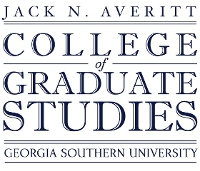## Electronic Theses and Dissertations

Fall 2012

#### Degree Name

Master of Science in Mathematics (M.S.)

#### Document Type and Release Option

Thesis (open access)

#### Department

Department of Mathematical Sciences

Colton Magnant

Andrew Sills

Hua Wang

Hua Wang

#### Abstract

In this thesis, we examine the use of integers through two ideas. As mathematics teachers, we prefer students not use calculators on assessments. In order to require this, students compute the problems by hand. We take a look at the classic Calculus I optimization box problem while restricting values to integers. In addition, sticking with the integer theme, we take a new look at the nexus numbers. Nexus numbers are extensions of the hex and rhombic dodecahedral numbers. We put these numbers into a sequence, and through a few computations of modular arithmetic, we analyze the sequences and their patterns based upon the different moduli. These patterns are specific to whether the power is even or odd. Within each power, there are other properties to this set of sequences. Depending on modulus, there are some sequences that stand out more than others.

No

COinS